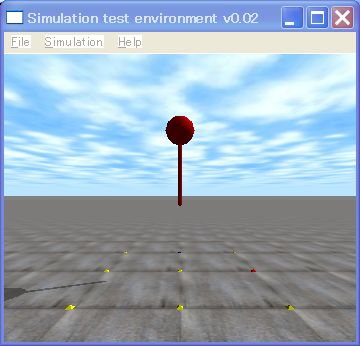# 7. JointThis is the 7th ODE (Open Dynamics Engine) tutorial.

This tutorial explains a joint.

There are a lot of joints around us. For example, a hinge of a cellular phone,  a hinge of a door, and so on.  A joint connect two bodies.  In other words, a joint is a constraint which restricts a movement of two bodies.  ODE uses a joint in the same meaning of a constraint.

• How to use a joint
1. Create a joint
• dJointCreate***()
2. connect bodies with the joint
• dJointAttach ()
3. Set an anchor of the joint
• dJointSet*** Anchor()
4. Set an axis of the joint
• dJointSet***

*** means a type of joint, such as a Hinge, Ball, Slider, Universal, and so non. Please read theUser Manual of ODE.

• How to set parameters of the joint
1. Set range of motion
dJointSetHingeParam (dJointID, dParamLoStop, the minimum range of motion);
dJointSetHingeParam (dJointID, dParamHiStop, the maximum range of motion);
2. Set angular velocity and maximum torque
dJointSetHingeParam (dJointID, dParamVel, target angular velocity);
dJointSetHingeParam (dJointID, dParamFMax, maximum torque);

You can set parameters of a joint. dParamLoStop is the minimum and dParamHiStop is the maximum range of motion. dParamVelis target speed and dParamFMaxis maximum torque to apply the joint.

• Sample program 7

Now,  a sample program of a hopping robot (above the figure)  is shown as follows.

```// sample7.cpp  by Kosei Demura 2006-2009
#ifdef  dDOUBLE
#define dsDrawSphere dsDrawSphereD
#define dsDrawCapsule  dsDrawCapsuleD
#endif

typedef struct {
dBodyID body;   // a body
dGeomID geom;　//  a geometry

static void simLoop (int pause)
{
const dReal *pos1,*R1,*pos2,*R2;
dSpaceCollide(space,0,&nearCallback); 　// Collision detection
dWorldStep(world,0.01);　// Step a world for 0.01 [sec]
dJointGroupEmpty(contactgroup);  // Empty the joint group

// Draw a ball
dsSetColor(1.0,0.0,0.0);   // Set red color
pos1 = dBodyGetPosition(ball.body);　// Get a position
R1   = dBodyGetRotation(ball.body);　// Get an orientation

// Draw a capsule
pos2 = dBodyGetPosition(pole.body);　// Get a position
R2   = dBodyGetRotation(pole.body);　// Get an orientation
}

// Create an object
void createBallPole()
{
dMass m1, m2;
dReal x0 = 0.0, y0 = 0.0, z0 = 2.5;    // a ball
ball.mass   = 1.0;
ball.body = dBodyCreate(world);
dMassSetZero(&m1);
dBodySetMass(ball.body,&m1);
dBodySetPosition(ball.body, x0, y0, z0);
dGeomSetBody(ball.geom,ball.body);

// a capsule
pole.length = 1.0;
pole.mass   = 1.0;
pole.body =  dBodyCreate(world);
dMassSetZero(&m2);
dBodySetMass(pole.body,&m2);
dBodySetPosition(pole.body, x0, y0, z0 - 0.5 * pole.length);
dGeomSetBody(pole.geom,pole.body);

// hinge joint
joint = dJointCreateHinge(world, 0);　// Create a hinge joint
dJointAttach(joint, ball.body,pole.body);　// Attach joint to bodies
dJointSetHingeAnchor(joint, x0, y0, z0 - ball.radius);　 // Set a joint anchor
dJointSetHingeAxis(joint, 1, 0, 0);　// Set a hinge axis(1,0,0)
}

int main (int argc, char **argv)
{
setDrawStuff();　　// Set a draw function
dInitODE();       // Initialize ODE
world = dWorldCreate();　// Create a world
space = dHashSpaceCreate(0);　// Create a collision space
contactgroup = dJointGroupCreate(0);　// Create a joint group
dWorldSetGravity(world,0,0,-9.8);  // Set gravity
ground = dCreatePlane(space,0,0,1,0); // Create a ground
createBallPole();　// Create ball and capsule

dsSimulationLoop (argc,argv,352,288,&fn);  // Simulation loop

dWorldDestroy (world);　// Destroy the world
dCloseODE();  // Close ODE
return 0;
}```

In this example, a robot is composed of a hinge joint which connects a torso and a leg.

Let’s read the source code. createBallandPolefunction is to create a ball for a torso , and a cylinder for a leg, and create a joint by  dJointCreateHinge().Bodies were connected  with the joint by  dJointAttach().

Then, dSetHingeAnchor() sets an anchor of the joint and dJointSetHingeAxis() sets an axis of the hinge joint. This example, the rotation axis vector is set to (1,0,0), i.e.,  the axis vector is the x-axis.

I did not set parameters of the joints  to simplify  the  source code. Usually, you can set many parameters about joints. Please see the ODE user manual in detail.

See you !

demu

APIs

• void dJointAttach (dJointID joint, dBodyID body1, dBodyID dBody2)
Attach the joint between body1 and body2
• dJointID dJointCreateHinge (dWorldID world, dJointGroupID)
Create a hinge Joint. dJointGroupID is set to zero.
• void dJointSetHingeAnchor (dJointID joint, dReal x, dReal y, dReal z)
Set an anchor point (x, y, z) of the hinge joint.

• void dJointSetHingeAxis (dJointID joint, dReal x, dReal y, dReal z)
Set an axis vector (x, y, z) of the hinge joint.

スポンサーリンク
demura.netをフォローするdemura.net

### Folding@home Kanazawa (ID 257261)

みんなのためにおうちで新型コロナウイルスを解析しよう！

タイトルとURLをコピーしました